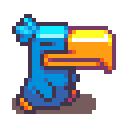Author Topic: Bounding box and angle  (Read 1447 times)

michelsteege

• New Member
•• Posts: 4
• Karma: +0/-0Bounding box and angle
« on: Tue, Dec 6, 2011 »
Hi all,

First an image to display my problem:I have a tank and a tilemap, you can drive the tank, but when you turn the tank obviously changes the angle this is all working but the problem is when i add collision between the tank and the tilemap when i change the angle of the tank the bounding box does not change.
Is this a bug in flixel or is this not supported?

I tried changing the bounding box myself by setting the width and height of the tank, i can get it in the correct size but not at the correct position i used this code:

Code: [Select]
var m_HalfSizeX:Number = 19;
var m_HalfSizeY:Number = 40;
var sin_o:Number = Math.sin(_base.angle * -0.017453293);
var cos_o:Number = Math.cos(_base.angle * -0.017453293);
var xformed_corner_1_x:Number = m_HalfSizeX * cos_o - (-m_HalfSizeY) * sin_o;
var xformed_corner_1_y:Number = m_HalfSizeX * sin_o + (-m_HalfSizeY) * cos_o;
var xformed_corner_2_x:Number = m_HalfSizeX * cos_o - m_HalfSizeY * sin_o;
var xformed_corner_2_y:Number = m_HalfSizeX * sin_o + m_HalfSizeY * cos_o;
var ex:Number = Math.max(Math.abs(xformed_corner_1_x), Math.abs(xformed_corner_2_x));
var ey:Number = Math.max(Math.abs(xformed_corner_1_y), Math.abs(xformed_corner_2_y));
_base.width = (_base.x + ex) - (_base.x - ex);
_base.height = (_base.y + ey) - (_base.y - ey);

I hope someone has a solution for this!

camasthecatRe: Bounding box and angle
« Reply #1 on: Tue, Dec 6, 2011 »
Try PhotonStorm's pixel perfect check.photonstorm

• Key Contributor
••• Posts: 1502
• Karma: +1/-0Re: Bounding box and angle
« Reply #2 on: Tue, Dec 6, 2011 »
Sorry Flixel only supports bounding boxes in the way you've shown, it can't handle rotation of them and still process collision / separation. You'll need to find another solution to get the same result I fear.
http://www.photonstorm.com"Tell me and I will forget, show me and I might remember, involve me and I will understand" - Confucius

michelsteege

• New Member
•• Posts: 4
• Karma: +0/-0Re: Bounding box and angle
« Reply #3 on: Wed, Dec 7, 2011 »
@camasthecat that one does not work with the tilemap@photonstorm hmm ok to bad! i will use a different framework for this game and come back to flixel latercamasthecatRe: Bounding box and angle
« Reply #4 on: Wed, Dec 7, 2011 »
Quote
@camasthecat that one does not work with the tilemap

Ah. I forgot.